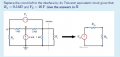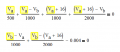# Thévenin equivalent circuit homework help

#### Artëm “Artjom” Dembski

Joined Sep 28, 2015
8
I have some issues with solving this Thévenin Equivalent Circuit homework assignment.
I know the steps I need to take to finish this assignment but when calculating the Thévenin Voltage, I get confused by the Vs. To which nodes does this voltage apply and how many calculations do I need to find the resulting Thévenin voltage?The Thévenin resistance is 333.33 Ohm. (Already calculated it)

#### Jony130

Joined Feb 17, 2009
5,176
Simply use any network analysis technique you know and find the voltage across 1K (on the right side of a diagram) resistor.

#### Artëm “Artjom” Dembski

Joined Sep 28, 2015
8
Simply use any network analysis technique you know and find the voltage across 1K (on the right side of a diagram) resistor.
I understand that. I just do not know how to deal with the Vs voltage over the 2 nodes.

If I apply KVL (Vx is the voltage to be calculated for the Thévenin Voltage),
(Vb-Va)/1k - 4mA + (Vb-Vx)/2k = 0
(Vx-Vb)/2k + Vx/1k + Vx - Vs???? I don't know how to deal with the Vs.

#### Artëm “Artjom” Dembski

Joined Sep 28, 2015
8
Vs = 16V but there is no resistor between node A and node X so they are both 16V right?
But 16V is not the Thévenin resistance

#### WBahn

Joined Mar 31, 2012
25,906
I have some issues with solving this Thévenin Equivalent Circuit homework assignment.
I know the steps I need to take to finish this assignment but when calculating the Thévenin Voltage, I get confused by the Vs. To which nodes does this voltage apply and how many calculations do I need to find the resulting Thévenin voltage?
The Vs supply establishes a voltage of Vs between the two nodes that it is connected to. That's what a voltage supply does.

#### WBahn

Joined Mar 31, 2012
25,906
I understand that. I just do not know how to deal with the Vs voltage over the 2 nodes.

If I apply KVL (Vx is the voltage to be calculated for the Thévenin Voltage),
(Vb-Va)/1k - 4mA + (Vb-Vx)/2k = 0
(Vx-Vb)/2k + Vx/1k + Vx - Vs???? I don't know how to deal with the Vs.
(Vx-Vb)/2k + Vx/1k + Vx - Vs

You KNOW that this is incorrect because the first two terms are currents (voltage divided by resistance) while the last two terms are voltages. You can add currents and voltages.

#### Artëm “Artjom” Dembski

Joined Sep 28, 2015
8
The Vs supply establishes a voltage of Vs between the two nodes that it is connected to. That's what a voltage supply does.
I understand. But how can I apply Vs in my calculations?

#### Jony130

Joined Feb 17, 2009
5,176
Well you have a super node in your circuit, also notice that Vx = Va + Vs.
But do not forget that you always can can change the GND position into node Va, use a superposition to find Vx, or use a mesh analysis.

#### WBahn

Joined Mar 31, 2012
25,906
Vs = 16V but there is no resistor between node A and node X so they are both 16V right?
But 16V is not the Thévenin resistance
I assume that Node X is the top right node? It's not labeled and so you are making us guess.

You have a 16 V voltage source connected between two nodes, A and X. Does it make any sense at all to conclude that those two nodes are at the same voltage?

The Vs supply does what all (ideal) voltage supplies do -- they establish a specific voltage difference between the two nodes they are connected to. That means that (Vx-Va)=Vs.

#### Artëm “Artjom” Dembski

Joined Sep 28, 2015
8
I assume that Node X is the top right node? It's not labeled and so you are making us guess.

You have a 16 V voltage source connected between two nodes, A and X. Does it make any sense at all to conclude that those two nodes are at the same voltage?

The Vs supply does what all (ideal) voltage supplies do -- they establish a specific voltage difference between the two nodes they are connected to. That means that (Vx-Va)=Vs.
Yes, it is the top right node, apologies.

How can I use Vx-Va in the equation?

#### WBahn

Joined Mar 31, 2012
25,906
The first step is to be methodical and show us the loop/node equations that you are starting from. Let's make sure that you have them correct.

#### Artëm “Artjom” Dembski

Joined Sep 28, 2015
8
I just gave them:

Va/0.5k + (Va-Vb)/1k = 0
(Vb-Va)/1k - 4 mA + (Vb-Vx)/2K = 0
(Vx-Vb)/2k + Vx/1k = 0

#### WBahn

Joined Mar 31, 2012
25,906
I just gave them:

Va/0.5k + (Va-Vb)/1k = 0
(Vb-Va)/1k - 4 mA + (Vb-Vx)/2K = 0
(Vx-Vb)/2k + Vx/1k = 0
I don't see where you gave these before, but thanks for providing them here.

This is a good place to start because two of the three are wrong, so no point going any further.

Remember that nodal analysis is nothing more than a systematic way of applying KCL. So your first equation represents KCL applied at Node A. Your first term is the current flowing downward through Rx and your second is the current flowing to the right from Node A to Node B. What about the third path by which current can either enter or leave that node. An identical issue exists with your third equation.

The problem with applying KCL at a node connected to a voltage source is that there is usually no trivial way to develop an expression for the current flowing in it.

There are a couple of ways to tackle the problem. The first, as noted by Jony130, is to recognize that you are free to pick whatever node you want to as your reference ("ground"). If you pick Node A as your ground, then you know what the voltage on Node X is. Now you just need to find the voltage on Node B and Node G (the former ground node).

The other way is to group Node A and Node X into a supernode and apply KCL to the node, noting that Vx = Va + Vs.

#### Jony130

Joined Feb 17, 2009
5,176
If you have a supernode in your circuit you must treat it as if you had a one big node (supernode) in your circuit.
So to write a KCL you need to "short" Va node together with Vx node.
Watch this example

But why for example you do not use a superposition to solve this circuit if you have problem with the nodal analysis ?

#### RBR1317

Joined Nov 13, 2010
550

#### WBahn

Joined Mar 31, 2012
25,906
One can write the node equation for a supernode directly, just as for any other node. The only difference is that not all parts of the supernode are at the same voltage. This circuit has one supernode and one regular node (as shown in the diagram, the supernode is highlighted), so only two node equations are necessary.
View attachment 92229
The goal is to guide the person, not do their work for them. They need to struggle with concepts they are having difficulty with. Figure that they have seen these techniques done in class and in examples in the text and yet something isn't clicking. So having someone turn their homework into yet another worked example isn't likely to make things magically click.

#### RBR1317

Joined Nov 13, 2010
550
The goal is to guide the person, not do their work for them.
Yes, I had reservations about this; however I have seen too many explanations that just fail to show the conceptual simplicity of writing the node equation for the supernode, and also being able to maintain the procedural simplicity of having every term in the node equation begin with that node's voltage (as highlighted below). The object was to illustrate the simplicity of writing the supernode equation directly, and it did not seem appropriate to leave it at "It's so simple. You figure it out." At least I did delay posting to provide for a day "to struggle with concepts they are having difficulty with." Should I have waited longer?#### WBahn

Joined Mar 31, 2012
25,906
Hard to say -- and what amount of help is optimal and what is too much and what is not enough is always up for debate and varies from person to person in ways that are seldom easy to discern.

On a separate note -- it would be good if your work used units properly.

You can't add 16 to Va because Va is a voltage and 16 is just a number. Then Va/500 is still a voltage, it is not a current.

$$\frac{V_a}{500 \: \Omega} \; + \; \frac{V_a \: - \: V_b}{1000 \: \Omega} \; + \; \frac{V_a \: + \: 16 \: V}{1000 \: \Omega} \; + \; \frac{\(V_a \: + \: 16 \: V$$ \: - \: V_b}{2000 \: \Omega} \; = \; 0
\)

#### RBR1317

Joined Nov 13, 2010
550
On a separate note -- it would be good if your work used units properly.
It would be good, but it's not going to happen. That image is a screen capture from the symbolic algebra engine that I use. I'm not inclined to redo it with the equation editor in Libre Office. And the last time I tried TeX the preview mode would not show the equation image. Was I doing something wrong, or is the preview not capable of showing a TeX preview?

#### WBahn

Joined Mar 31, 2012
25,906
It would be good, but it's not going to happen. That image is a screen capture from the symbolic algebra engine that I use. I'm not inclined to redo it with the equation editor in Libre Office. And the last time I tried TeX the preview mode would not show the equation image. Was I doing something wrong, or is the preview not capable of showing a TeX preview?
I understand the first motive -- it's definitely an unfortunate shortcoming of may math packages. The preview mode is working fine for TeX. It might have been having issues the particular time that you tried to use it. Also, if there is an error in your LaTeX code the rendering results can be pretty unpredictable, including not rendering anything at all. It can make it a pain to track down the problem.